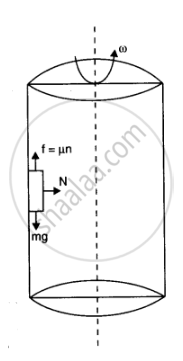CBSE (Science) Class 11CBSE
Share

# A 70 Kg Man Stands in Contact Against the Inner Wall of a Hollow Cylindrical Drum of Radius 3 M Rotating About Its Vertical Axis with 200 Rev/Min. the Coefficient of Friction Between the Wall and His Clothing is 0.15. What is the Minimum Rotational Speed of the Cylinder to Enable the Man to Remain Stuck to the Wall (Without Falling) When the Floor is Suddenly Removed - CBSE (Science) Class 11 - Physics

#### Question

A 70 kg man stands in contact against the inner wall of a hollow cylindrical drum of radius 3 m rotating about its vertical axis with 200 rev/min. The coefficient of friction between the wall and his clothing is 0.15. What is the minimum rotational speed of the cylinder to enable the man to remain stuck to the wall (without falling) when the floor is suddenly removed?

#### Solution 1

Mass of the man, m = 70 kg

Radius of the drum, r = 3 m

Coefficient of friction, μ = 0.15

Frequency of rotation, ν = 200 rev/min = 200/60 = 10/3 "rev/s"

The necessary centripetal force required for the rotation of the man is provided by the normal force (FN).

When the floor revolves, the man sticks to the wall of the drum. Hence, the weight of the man (mg) acting downward is balanced by the frictional force
(μFN) acting upward.

Hence, the man will not fall until:

mg < f

mg < μFN = μmrω2

g < μrω2

The minimum angular speed is given as:

omega_"min"= sqrt(g/mu r)

= sqrt(10/(0.15 xx 3)) = 4.71 "rad s"^(-1)

#### Solution 2

R = 3m, omega = 200 rev/min = 2xx22/7xx200/60 "rad/s" = 440/21 "rad/s" and mu  = 0.15

As shown in the figure, the normal reaction (N) of the wall on the man acts in the horizontal direction towards the axis of the cylinder while the force of friction (f) acts vertically upwards. The required centripetal force will be provided by the horizontal reaction N of the wall on the man i.e,

N  = (mv^2)/R = momega^2R

The frictional force f acting vertically upwards will be balanced by the weight of the man. Hence, the man remains stuck to the wall after the floor is removed if mg ≤ limiting frictional force f_e  (or muN)

or if mg <= mu m omega^2R

or g <= mu omega^2 R

or mu omega^2 R >= g or omega >= g/(Rmu)

Hence, for minimum rotational speed of the cylinder

omega^2 =  g/(muR) = 10/(0.15 xx 3) = 22.2

=>omega =  sqrt(22.2) = 4.7 rad/sIs there an error in this question or solution?

#### APPEARS IN

Solution A 70 Kg Man Stands in Contact Against the Inner Wall of a Hollow Cylindrical Drum of Radius 3 M Rotating About Its Vertical Axis with 200 Rev/Min. the Coefficient of Friction Between the Wall and His Clothing is 0.15. What is the Minimum Rotational Speed of the Cylinder to Enable the Man to Remain Stuck to the Wall (Without Falling) When the Floor is Suddenly Removed Concept: Circular Motion.
S